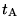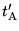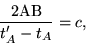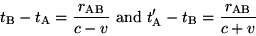# On the Electrodynamics of Moving Bodies

## Annalen der Physik, 17 (1905), pp. 891-921

### Albert Einstein

(Introduction, Sections 1 and 2 only. For an online version of the complete text, see
http://www.fourmilab.ch/etexts/einstein/specrel/www/, but beware of the error noted below. This page is drawn from this online source.)

It is known that Maxwell's electrodynamics--as usually understood at the present time--when applied to moving bodies, leads to asymmetries which do not appear to be inherent in the phenomena. Take, for example, the reciprocal electrodynamic action of a magnet and a conductor. The observable phenomenon here depends only on the relative motion of the conductor and the magnet, whereas the customary view draws a sharp distinction between the two cases in which either the one or the other of these bodies is in motion. For if the magnet is in motion and the conductor at rest, there arises in the neighbourhood of the magnet an electric field with a certain definite energy, producing a current at the places where parts of the conductor are situated. But if the magnet is stationary and the conductor in motion, no electric field arises in the neighbourhood of the magnet. In the conductor, however, we find an electromotive force, to which in itself there is no corresponding energy, but which gives rise--assuming equality of relative motion in the two cases discussed--to electric currents of the same path and intensity as those produced by the electric forces in the former case.

Examples of this sort, together with the unsuccessful attempts to discover any motion of the earth relatively to the light medium,'' suggest that the phenomena of electrodynamics as well as of mechanics possess no properties corresponding to the idea of absolute rest. They suggest rather that, as has already been shown to the first order of small quantities, the same laws of electrodynamics and optics will be valid for all frames of reference for which the equations of mechanics hold good.1 We will raise this conjecture (the purport of which will hereafter be called the Principle of Relativity'') to the status of a postulate, and also introduce another postulate, which is only apparently irreconcilable with the former, namely, that light is always propagated in empty space with a definite velocity c which is independent of the state of motion of the emitting body. These two postulates suffice for the attainment of a simple and consistent theory of the electrodynamics of moving bodies based on Maxwell's theory for stationary bodies. The introduction of a luminiferous ether'' will prove to be superfluous inasmuch as the view here to be developed will not require an absolutely stationary space'' provided with special properties, nor assign a velocity-vector to a point of the empty space in which electromagnetic processes take place.

The theory to be developed is based--like all electrodynamics--on the kinematics of the rigid body, since the assertions of any such theory have to do with the relationships between rigid bodies (systems of co-ordinates), clocks, and electromagnetic processes. Insufficient consideration of this circumstance lies at the root of the difficulties which the electrodynamics of moving bodies at present encounters.

# I. KINEMATICAL PART

## § 1. Definition of Simultaneity

Let us take a system of co-ordinates in which the equations of Newtonian mechanics hold good.2 In order to render our presentation more precise and to distinguish this system of co-ordinates verbally from others which will be introduced hereafter, we call it the stationary system.''

If a material point is at rest relatively to this system of co-ordinates, its position can be defined relatively thereto by the employment of rigid standards of measurement and the methods of Euclidean geometry, and can be expressed in Cartesian co-ordinates.

If we wish to describe the motion of a material point, we give the values of its co-ordinates as functions of the time. Now we must bear carefully in mind that a mathematical description of this kind has no physical meaning unless we are quite clear as to what we understand by time.'' We have to take into account that all our judgments in which time plays a part are always judgments of simultaneous events. If, for instance, I say, That train arrives here at 7 o'clock,'' I mean something like this: The pointing of the small hand of my watch to 7 and the arrival of the train are simultaneous events.''3

It might appear possible to overcome all the difficulties attending the definition of time'' by substituting the position of the small hand of my watch'' for time.'' And in fact such a definition is satisfactory when we are concerned with defining a time exclusively for the place where the watch is located; but it is no longer satisfactory when we have to connect in time series of events occurring at different places, or--what comes to the same thing--to evaluate the times of events occurring at places remote from the watch.

We might, of course, content ourselves with time values determined by an observer stationed together with the watch at the origin of the co-ordinates, and co-ordinating the corresponding positions of the hands with light signals, given out by every event to be timed, and reaching him through empty space. But this co-ordination has the disadvantage that it is not independent of the standpoint of the observer with the watch or clock, as we know from experience. We arrive at a much more practical determination along the following line of thought.

If at the point A of space there is a clock, an observer at A can determine the time values of events in the immediate proximity of A by finding the positions of the hands which are simultaneous with these events. If there is at the point B of space another clock in all respects resembling the one at A, it is possible for an observer at B to determine the time values of events in the immediate neighbourhood of B. But it is not possible without further assumption to compare, in respect of time, an event at A with an event at B. We have so far defined only an A time'' and a B time.'' We have not defined a common time'' for A and B, for the latter cannot be defined at all unless we establish by definition that the time'' required by light to travel from A to B equals the time'' it requires to travel from B to A. [JDN: this last sentence is a notorious misrendering in the standard edition of Einstein's original text. It should read "...and the latter can now be determined by establishing by definition that the "time" needed for the light to travel from A to B is equal to the "time" it needs to travel from B to A.] Let a ray of light start at the A time''from A towards B, let it at the B time''be reflected at B in the direction of A, and arrive again at A at the A time''.

In accordance with definition the two clocks synchronize ifWe assume that this definition of synchronism is free from contradictions, and possible for any number of points; and that the following relations are universally valid:--

1. If the clock at B synchronizes with the clock at A, the clock at A synchronizes with the clock at B.
2. If the clock at A synchronizes with the clock at B and also with the clock at C, the clocks at B and C also synchronize with each other.

Thus with the help of certain imaginary physical experiments we have settled what is to be understood by synchronous stationary clocks located at different places, and have evidently obtained a definition of simultaneous,'' or synchronous,'' and of time.'' The time'' of an event is that which is given simultaneously with the event by a stationary clock located at the place of the event, this clock being synchronous, and indeed synchronous for all time determinations, with a specified stationary clock.

In agreement with experience we further assume the quantityto be a universal constant--the velocity of light in empty space.

It is essential to have time defined by means of stationary clocks in the stationary system, and the time now defined being appropriate to the stationary system we call it the time of the stationary system.''

## § 2. On the Relativity of Lengths and Times

The following reflexions are based on the principle of relativity and on the principle of the constancy of the velocity of light. These two principles we define as follows:--

1. The laws by which the states of physical systems undergo change are not affected, whether these changes of state be referred to the one or the other of two systems of co-ordinates in uniform translatory motion.
2. Any ray of light moves in the stationary'' system of co-ordinates with the determined velocity c, whether the ray be emitted by a stationary or by a moving body. Hencewhere time interval is to be taken in the sense of the definition in § 1.

Let there be given a stationary rigid rod; and let its length be l as measured by a measuring-rod which is also stationary. We now imagine the axis of the rod lying along the axis of x of the stationary system of co-ordinates, and that a uniform motion of parallel translation with velocity v along the axis of x in the direction of increasing x is then imparted to the rod. We now inquire as to the length of the moving rod, and imagine its length to be ascertained by the following two operations:--

(a)
The observer moves together with the given measuring-rod and the rod to be measured, and measures the length of the rod directly by superposing the measuring-rod, in just the same way as if all three were at rest.
(b)
By means of stationary clocks set up in the stationary system and synchronizing in accordance with § 1, the observer ascertains at what points of the stationary system the two ends of the rod to be measured are located at a definite time. The distance between these two points, measured by the measuring-rod already employed, which in this case is at rest, is also a length which may be designated the length of the rod.''

In accordance with the principle of relativity the length to be discovered by the operation (a)--we will call it the length of the rod in the moving system''--must be equal to the length l of the stationary rod.

The length to be discovered by the operation (b) we will call the length of the (moving) rod in the stationary system.'' This we shall determine on the basis of our two principles, and we shall find that it differs from l.

Current kinematics tacitly assumes that the lengths determined by these two operations are precisely equal, or in other words, that a moving rigid body at the epoch t may in geometrical respects be perfectly represented by the same body at rest in a definite position.

We imagine further that at the two ends A and B of the rod, clocks are placed which synchronize with the clocks of the stationary system, that is to say that their indications correspond at any instant to the time of the stationary system'' at the places where they happen to be. These clocks are therefore synchronous in the stationary system.''

We imagine further that with each clock there is a moving observer, and that these observers apply to both clocks the criterion established in § 1 for the synchronization of two clocks. Let a ray of light depart from A at the time4, let it be reflected at B at the time, and reach A again at the time. Taking into consideration the principle of the constancy of the velocity of light we find thatwheredenotes the length of the moving rod--measured in the stationary system. Observers moving with the moving rod would thus find that the two clocks were not synchronous, while observers in the stationary system would declare the clocks to be synchronous.

So we see that we cannot attach any absolute signification to the concept of simultaneity, but that two events which, viewed from a system of co-ordinates, are simultaneous, can no longer be looked upon as simultaneous events when envisaged from a system which is in motion relatively to that system.

#### Footnotes (in the Teubner edition)

1.
The preceding memoir by Lorentz was not at this time known to the author.
2.
i.e. to the first approximation.
3.
We shall not here discuss the inexactitude which lurks in the concept of simultaneity of two events at approximately the same place, which can only be removed by an abstraction.
4.
Time'' here denotes time of the stationary system'' and also position of hands of the moving clock situated at the place under discussion.''